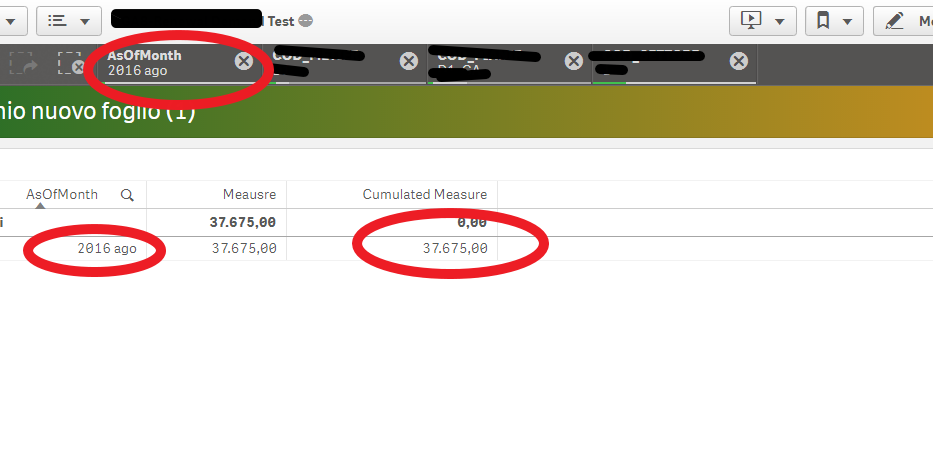New to Qlik Sense

Discussion board where members can get started with Qlik Sense.Partner

Dynamic Set Analysis

Hi All,

i've an issue with Set Analysis.

I have to calculate a Cumulative measure but i cannot use above function because it doesn't work correctly for me when i make selections.

The reference measure is

Sum( {\$< MonthDiff={'-1'} >} #MEASURE)

i'm trying to use this formula for having cumulate:

Sum( {\$< MonthDiff={'-1'} >} #MEASURE)  +

Sum({\$<MonthDiff={">=0 <=\$(=num(Month(AsOfMonth))-2)"}  #MEASURE)

The problem is that the string    <=\$(=num(Month(AsOfMonth))-2)    doesn't work.

How can i write this for Set Analysis?

Thank you All.

Tags (2)
11 Replies
Honored Contributor III

Re: Dynamic Set Analysis

What is the value in AsOfMonth?

If you put the expression num(Month(AsOfMonth))-2

in a text box, do you get the numeric value?MVP

Re: Dynamic Set Analysis

You might be able to make Above() work even with selections.... do you have an example where Above() is not working with selections?Partner

Re: Dynamic Set Analysisif i make selection:Partner

Re: Dynamic Set Analysis

For me The AsOfTable is a Time field 'YYYY MMM'MVP

Re: Dynamic Set Analysis

What was the expression you used for accumulation?Partner

Re: Dynamic Set Analysis

Rangesum( Above(Sum({\$<MonthDiff={'-1'}>}  #MEASURE ) ,0, RowNo(total)))MVP

Re: Dynamic Set Analysis

Try this

Rangesum(Above(Sum({\$<MonthDiff={'-1'}, AsOfMonth>}  #MEASURE ) ,0, RowNo(total))) * Avg({\$<MonthDiff={'-1'}>} 1)Partner

Re: Dynamic Set Analysis

Conceptually it is right and works.

But isn't what i'm looking for.MVP

Re: Dynamic Set Analysis

oh okay... but if it gives the right number, then why are you not looking for this